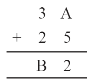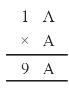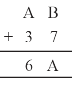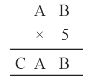# NCERT Solutions for Class 8 Maths Chapter 16 Playing with Numbers| PDF Download

Chapter 16 Playing with Numbers Class 8 Maths NCERT Solutions will help you in knowing the latest pattern of CBSE and developing problem solving skills. Chapter 16 NCERT Solutions for Class 8 Maths PDF can be downloaded from here which help in preparing for the exams with ease. These solutions are updated according to the latest NCERT Class 8 Maths textbook.

Class 8 Maths is good way through which one can build their basic knowledge and getting good marks in the examination. It will be really useful in completing homework on time and scoring great marks in the exams.Page No: 220

Exercise 16.1

Find the values of the letters in each of the following and give reasons for the steps involved.

1.On putting A = 1, 2, 3, 4, 5, 6, 7 and so on and we get, 7 + 5 = 12 in which ones place is 2.
∴ A = 7
And putting 2 and carry over 1, we get
B = 6
Hence A = 7 and B = 6

2.On putting A = 1, 2, 3, 4, 5, 6, 7 and so on and we get,8 + 5 = 13 in which ones place is 3.
∴ A = 5
And putting 3 and carry over 1, we get
B = 4 and C = 1
Hence A = 5, B = 4 and C = 1

3.On putting A = 1, 2, 3, 4, 5, 6, 7 and so on and we get, A x A = 6 x 6 = 36 in which ones place is 6.
∴ A = 6
Hence A = 6

4.Here, we observe that B = 5
So that 7 + 5 = 12.
Putting 2 at ones place and carry over 1 and A = 2, we get
2 + 3 + 1 = 6
Hence A = 2 and B = 5

5.Here on putting B = 0,
we get 0×3 = 0.
And A = 5, then 5×3 = 15
⇒ A = 5 and C = 1
Hence A = 5, B = 0 and C = 1

6.On putting B = 0, we get 0 , and A = 5, then 5× 5 = 25
⇒ A = 5, C = 2
Hence A = 5, B = 0 and C = 2

7.Here product of B and 6 must be same as ones place digit as B.
6 × 1 = 6, 6 × 2 = 12, 6 × 3 = 18,
6 × 4 = 24
On putting B = 4, we get the ones digit 4 and remaining two B’s value should be 44.
∴ For 6 × 7 = 42 and carry over 2 = 44
Hence A = 7 and B = 4

8.On putting B = 9, we get 9 + 1 = 10
Putting 0 at ones place and carry over 1, we get
For A = 7 ⇒ 7 + 1 + 1 = 9
Hence A = 7 and B = 9

9.On putting B = 7,
⇒ 7 + 1 = 8
Now A = 4, then 4 + 7 = 11
Putting 1 at tens place and carry over 1, we get
2 + 4 + 1 = 7
Hence A = 4 and B = 7

10.Putting A = 8 and B = 1, we get
8 + 1 = 9
Now again we add 2 + 8 = 10
Tens place digit is ‘0’ and carry over 1.
Now 1 + 6 + 1 = 8 = A
Hence A = 8 and B = 1

Page No. 260

Exercise 16.2

1. If 21y5 is a multiple of 9, where y is a digit, what is the value of y?

Since 21y5 is a multiple of 9.
Therefore according to the divisibility rule of 9, the sum of all the digits should be a multiple of 9.
∴ 2+2+y+5= 8+y
⇒ 8+y = 9
⇒ y = 1
Since 21y5 is a multiple of 9.

2. If 31z5 is a multiple of 9, where z is a digit, what is the value of z? You will find that there are two answers for the last problem. Why is this so?

Since 31z5 is a multiple of 9.
Therefore according to the divisibility rule of 9, the sum of all the digits should be a multiple of 9.
∴ 3+1+z+5 = 9+z
⇒ 9+z = 9
⇒ z= 0
If 3 +1+z+5 = 9+z
⇒ 9+a = 18
⇒ z= 9
Hence, 0 and 9 are two possible answers.

3. If 24x is a multiple of 3, where x is a digit, what is the value of x?
(Since 24x is a multiple of 3, its sum of digits 6 + x is a multiple of 3; so 6 + x is one of these numbers: 0, 3, 6, 9, 12, 15, 18, ... But since x is a digit, it can only be that 6 + x = 6 or 9 or 12 or 15. Therefore, x = 0 or 3 or 6 or 9. Thus, x can have any of four different values.)

Since, 24x is a multiple of 3.
Therefore according to the divisibility rule of 3, the sum of all the digits should be a multiple of 3.
∴ 2+4+x = 6 +x
Since x is a digit.
⇒6+x= 6 ⇒ x= 0
⇒ 6+x = 9 ⇒ x= 3
⇒ 6+ x = 12 ⇒x= 6
⇒ 6+x = 15 ⇒ x = 9
Thus, x can have any of four different values.

4. If 31z5 is a multiple of 3, where z is a digit, what might be the values of z?

Since 31z5 is a multiple of 3.
Therefore according to the divisibility rule of 3, the sum of all the digits should be a multiple of 3.
Since z is a digit.
∴ 3+1+z+5 = 9+z
⇒ 9+z=9 ⇒ z= 0
If 3+1+z+5 = 9+z
⇒ 9 +z = 12 ⇒ z= 3
If 3+1+z+5 = 9+z
⇒ 9 + z= 15 ⇒ z= 6
If 3+1+z+5 = 9+z
⇒ 9 +z = 18 ⇒ z= 9
Hence 0, 3, 6 and 9 are four possible answers.

## NCERT Solutions for Class 8 Maths Chapter 16 Playing with Numbers

In the Chapter 16 NCERT Solutions, we will explore numbers in more detail and justify tests of divisibility.

• A number is divisible by 10, if its ones digits is 0.
• A number is divisible by 5, if its ones digits is 0 or 5.
• A number is divisible by 2, if its ones digits is 0, 2, 4, 6 or 8.
• A number is divisible by 9, if the sum of its digits is divisible by 9.
• A number is divisible by 3, if the sum of its digits is divisible by 3.

We have provided exercisewise NCERT Solutions for Class 8 below that will serve your need and become invaluable resource to tackle problems given in the chapter. These NCERT Solutions will give edge over classmates.

### NCERT Solutions for Class 8 Maths Chapters:

 Chapter 1 Rational Numbers Chapter 2 Linear Equations in Variable Chapter 3 Understanding Quadrilaterals Chapter 4 Practical Geometry Chapter 5 Data Handling Chapter 6 Squares and Square Roots Chapter 7 Cubes and Cube Roots Chapter 8 Comparing Quantities Chapter 9 Algebraic Expressions and Identities Chapter 10 Visualising Solid Shapes Chapter 11 Mensuration Chapter 12 Exponents and Powers Chapter 13 Direct and Inverse Proportions Chapter 14 Factorisation Chapter 15 Introduction to Graphs

FAQ on Chapter 16 Playing with Numbers

#### How many exercises are there in Chapter 9 Areas of Parallelograms and Triangles?

There are total 2 exercises in the chapter which will improve your skills and detailed every solutions step by step so you can easily know the concepts. It will help in solving the difficulties that lie ahead with ease.

#### If 27x is a multiple of 3 and x is a digit then find the value of x.

Sum of the digits of 27x
= 2 + 7 + x = 9 + x
Since, 27x is a multiple of 3,
∴ (9 + x) is divisible by 3.
⇒ 9 + x must be equal to 9 or 12 or 15
When 9 + x = 9 ⇒ x = 0
When 9 + x = 12 ⇒ x = 3
When 9 + x = 15 ⇒ x = 6
When 9 + x = 18 ⇒ x = 9
When 9 + x = 21 ⇒ x = 12, which is not possible. [∵ x is a digit]
The x can equal to 0, 3, 6 or 9.

#### If 42x5 is a multiple of 9 and x is a digit, then find the value of x.

x = 7 is the answer.

#### If 51×3 is a multiple of 9, where x is a digits, then what is the value of x?

We have the sum of the digits of 51x3
= 5 + 1 + x + 3 = 9 + x
Since, 51x3 is divisible by 9.
∴ (9 + x) must be divisible by 9.
∴ (9 + x) must be equal to 0 or 9 or 18 or 27 or …
But x is a digit, then
9 + x = 9 ⇒ x = 0
9 + x = 18 ⇒ x = 9
x = 27 ⇒ x = 18, which is not possible.
∴ The required value of x = 0 or 9.